# Distance Protection | Impedance Relay

Distance protection | Impedance relay is a widely used protective scheme for the protection of high or an extra high voltage transmission lines.

The operation of the conventional over current relays either direction or non directional, depend on the magnitude of current or power in the protected circuit, where as the distance protection relay operate on the principle of the ratio of applied voltage to current in that circuit. This ratio is proportional to the distance along the line, and the relay that measures the distance is called distance protection relay. It is not unit system of protection. A single scheme provides both primary and backup protections.

# Types of Distance Protection Relay

The distance protection relay family consists of the following types of Relays:

1. Impedance Relays
2. Reactance Relays
3. MHO Relays or Admittance Relays.

# Impedance Relay

An impedance relay measures the impedance of the line at the relay location. When a fault occurs in the protected line section the measured impedance is the impedance of the line section between the relay location and the point of fault. It is proportional to the length of the line and hence to the distance along the line as shown below.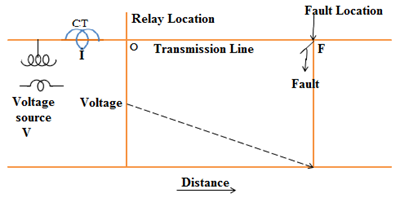OF is the distance along the line between the relay location and the fault location, The voltage drop along OF and the current I flowing in the line are taken for measurement by the relay and the ratio of the both quantities is nothing but impedance.

## Construction of Impedance Relay

The figure shows the simple arrangement of impedance relay operates based on the distance of the fault.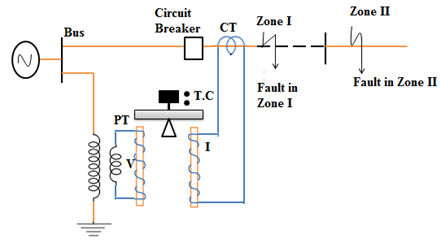Here, balanced beam type EM relay is used as impedance relay. The CT and PT are energized by the current and voltage of the circuit which is to be protected.

## Operating Principle of Impedance Relay

A simple form of EM balanced beam impedance relay is shown in the fig. It has a fixed beam and two electromagnets (EM). One EM is energized by voltage of the zone through PT and the other EM is energized by current of the zone through CT.

Under no fault condition, the pull due to voltage element will be more than the pull due to current element and the trip circuit (TC) remains open.

Since this type of relay operates based on the impedance of the circuit which in turn depends upon the distance of the fault from the relay location, it is called distance relay.

In operating characteristics of an impedance of the circuit is compared with voltage at relay location. The current produces a positive torque, called Operating Torque and the voltage produces a negative torque called Restraining Torque. This equation for the operating torque of an electromagnetic relay is:
T = K1 I2 – K2V2 – K3
Where K1, K2, K3 are constants, K3 being the torque due to control spring effect.

Neglecting the effect of the spring used, which is very small, the torque equation can be written as:
T = K1 I2 – K2V2
For the operation of the relay, the following condition should be satisfied.
K1I2 > K2V or   K2V2 < K1I2
V2/I2 < K1/K2

V/I < K where K is a constant.
as V/I is Z, Z<K.

The above expression explains that the relay is on the verge of operation when the ratio of V to I i.e., the measured value of line impedance is equal to a given constant. The relay operates, if the measured Z is less than the given constant. This given constant, is a design value depending on the total length of the HT / EHT feeder to be protected.

A distance relay can also be called as an Ohmmeter, measuring the impedance of the line in ohms.

## Operating Characteristic of an Impedance Relay (V-I and R-X Diagram)

#### V-I Diagram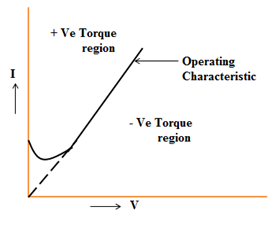The above figure shows the operating characteristic of impedance relay in terms of voltage and current. Hence the above is termed as V-I diagram. The operating characteristic is slightly bent near the origin due to the effect of the control spring. If the relay is of static relay type, the characteristic would have been a straight line, as there is no control spring in the relay. The positive torque region is the relay operating zone (above the characteristic curve) and the negative torque region below the curve is the relay non-operating zone.

#### R-X Diagram

Another and more useful way of representing the operating characteristic of the relay is an R-X diagram as shown below: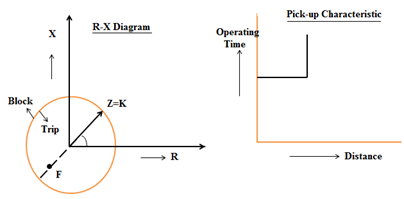Z = K = radius of the circle. When Z, i.e., the impedance of the line up to fault location measured from the relay location is less than K, the relay will operate i.e., the fault location lies inside the circle. If it is outside the circle, the relay will not sense it and hence, that zone is the Blocking zone. The operations of the relay depends upon the magnitude of Z and not on angle Φ, as Z is the radius of the circle, having equal magnitude along the circumference of the circle, from the centre. It is also seen that the impedance relay, basically a non-directional relay, as it operates on the magnitude of the operating quantity and not on its direction of flow, The figure indicates that the operating time of this relay is constant irrespective of the distance within the protective zone.

## Directional Impedance Relay

An impedance being non-directional in characteristic, will trip for a fault lying within the circle, irrespective, will trip of the fact that the fault point lies either in the forward direction or in the reverse direction. That is, the relay will also trip for the fault behind the relay location which is not a desirable one. To restrict the tripping zone in the forward direction only, a directional unit is included in the protective scheme.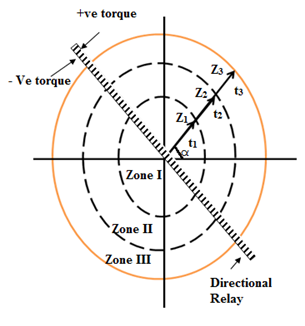At any relay location, three impedance relays and a directional unit connected in series with the impedance relays are provided.

The impedance of zone-I is Z1. If any fault occurs at F1, the impedance of zone-I reduce to a lower value than the pre-set value (Z1). Due to this the circuit increases and operates the relay.

For any fault at zone-II F2 in figure the impedance of zone-I will not change and the relay will no operate.

### Zones of Protection by Impedance Relay

Three units of impedance relays are normally required at a particular location for three zones of protection. It is the normal pratice to adjust the first unit to protect only up to 80% to 90% of the protected line. This 80% to 90% of the protected line is the first zone of protection. It is a high speed unit. Its operation is instantaneous about 1 to 2 cycle.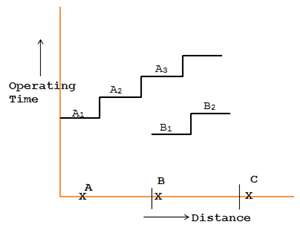The second unit protects the rest 20% of the protected line and 50% of the shortest adjoining line. This zone of protection is called second zone of protection. The second zone unit operates after a certain time delay. Its operating time is 0.2 to 0.5 sec.

The third unit is a back-up of the adjoining line. The setting of this unit covers the first line i.e., the protected line plus and longest second line plus 25% of the third line. The time of relay operation is 0.4 to 1 sec.

Because of the cost involved and relay panel space restriction, only one measuring unit is employed for all the three zones of protection nowadays. The timing unit sets the distance settings for II and III zones. The stepped time – distance characteristics of impedance relays are represented in the figure.

A1 A2 and A3 are the operating time for the zones 1, 2 and 3 relays placed at A. Similarly, B1, B2, B3 are the operating times for the zones 1, 2 and 3 relays placed at B.4 CommentsComments

#### Read Comment Policy We have Zero Tolerance to Spam. Chessy Comments and Comments with Links will be deleted immediately upon our review.

1.Need notes on Microprocessor based impedance relay

2.A framework utilizing OTP can be changed in accordance with suit a specific inclination or application. anti piracy security

3.Z-Wave is ideal for the Do-it-yourselfer. It's measured plan permits you to effectively include practically any gadget in minutes. nfc relay

4.Impedance relay direction or non directional